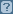# Date Transformations

In 1010data, dates are stored internally as 8-digit integers with the form YYYYMMDD. For instance, January 4, 2014, would be stored in 1010data as `20140104`. Though storing dates this way is more efficient in terms of processing speeds, this is not the way most of us are used to seeing dates. So 1010data uses display formats to show dates in more familiar forms. For example, `20140104` could be displayed as `01/04/14` using the `date` format, or as `01/04/2014` using the `date4y` format. Regardless of how this date is being displayed, under the covers it’s still being stored as the integer `20140104`.

However, sometimes dates are not loaded into 1010data in this integer format. Sometimes, dates are loaded as text values, and the forms that these strings can take may vary. Some examples are: `1/4/14`, `01042014`, `01-04-2014`, or `1/4/2014`. Dates stored as text are not recognized by 1010data as dates and can’t be used in any of the date-related functions. For instance, the function `year(X)` returns the year portion of a date value. However, if you try to provide this function with a date stored as text, you’ll get an error.

Note: You can find out the data type of the column containing your date values by clicking the question mark icon () at the top of the column.

The following are some examples of Macro Language code that perform some simple manipulations to transform your text columns into the proper integer data type so that they can be recognized and processed correctly in 1010data. All of the following transformations assume that the text column in which your dates are stored is named `date_column`, although that name is arbitrary and purely for example.

## MMDDYYYY

Example: 01042014

Note: The following transformation expects that the month consists of two characters, including the months January through September, which are represented by the two-digit values 01 through 09. If the text value of your date only has one digit values for these months, see below for the appropriate transformation.
``````<willbe name="new_date"
value="int(splice(strextract(date_column;5;4)
strextract(date_column;1;2) strextract(date_column;3;2);''))"
format="type:date"/>``````

## MM-DD-YYYY

Example: 01-04-2014

``````<willbe name="new_date"
value="int(splice(strpick(date_column;'-';3)
strpick(date_column;'-';1) strpick(date_column;'-';2);''))"
format="type:date"/>``````

## MM/DD/YYYY

Example: 01/04/2014

Note: The only difference between this example and the previous example is the delimiter character. Notice how the formulas are almost the same; the only difference is that the "`-`" has been replaced with "`/`". This same formula can be used regardless of delimiter, as long as similar character substitutions are made.
``````<willbe name="new_date"
value="int(splice(strpick(date_column;'/';3)
strpick(date_column;'/';1) strpick(date_column;'/';2);''))"
format="type:date"/>``````

## MM/DD/YY

Example: 01/04/14

``````<willbe name="new_date"
value="int(splice(splice('20' strpick(date_column;'/';3);'')
strpick(date_column;'/';1) strpick(date_column;'/';2);''))"
format="type:date"/>``````

## M/D/YYYY

Example: 1/4/2014

Note: This format will have various character widths since single-digit months and days drop the leading `0` and therefore will only have one character representing them instead of two. For this reason, our transformation must utilize the `if()` function.
``````<willbe name="new_date"
value="int(splice(strpick(date_column;'/';3)
if(width(strpick(date_column;'/';1))=1;
splice('0' strpick(date_column;'/';1);'');
strpick(date_column;'/';1))
if(width(strpick(date_column;'/';2))=1;
splice('0' strpick(date_column;'/';2);'');
strpick(date_column;'/';2));''))"
format="type:date"/>``````

## M/D/YY

Example: 1/4/14

Note: Similar to the previous example, this transformation will utilize the `if()` function because the width of the month and day portions can vary. The only difference is the year portion.
``````<willbe name="new_date"
value="int(splice(splice('20' strpick(date_column;'/';3);'')
if(width(strpick(date_column;'/';1))=1;
splice('0' strpick(date_column;'/';1);'');
strpick(date_column;'/';1))
if(width(strpick(date_column;'/';2))=1;
splice('0' strpick(date_column;'/';2);'');
strpick(date_column;'/';2));''))"
format="type:date"/>``````

## DD-Month-YY

Example: 04-Jan-14

In this case, instead of having numerical values representing the month, we have actual string names. Also, the values may not be in the most typical order, as the day appears before the month. This transformation will require some additional steps to first reorder the values, then replace all the names with numerical equivalents, and finally to do a transformation similar to the examples above.

``````<willbe name="date_reorder"
value="splice(strdrop(strtake(date_column;'-';2);'-';1)
strtake(date_column;'-';1) strdrop(date_column;'-';2);'-')"/>
<willbe name="date_replace" value="repstr(date_reorder;
'Jan' '01' 'Feb' '02' 'Mar' '03' 'Apr' '04' 'May' '05' 'Jun' '06'
'Jul' '07' 'Aug' '08' 'Sept' '09' 'Oct' '10' 'Nov' '11' 'Dec' '12')"/>
<willbe name="new_date"
value="int(splice(splice('20' strpick(date_replace;'-';3);'')
strpick(date_replace;'-';1) strpick(date_replace;'-';2);''))"
format="type:date"/>``````